# 31 Year NEET Previous Year Questions: Structure of Atom - 1

## 31 Questions MCQ Test Topic-wise MCQ Tests for NEET | 31 Year NEET Previous Year Questions: Structure of Atom - 1

Description
Attempt 31 Year NEET Previous Year Questions: Structure of Atom - 1 | 31 questions in 62 minutes | Mock test for NEET preparation | Free important questions MCQ to study Topic-wise MCQ Tests for NEET for NEET Exam | Download free PDF with solutions
QUESTION: 1

### The following quantum numbers are possible for how many orbital (s) n = 3, l = 2, m = +2 ? 

Solution:

Quantum number n = 3, l = 2, m = +2 represent an orbital  with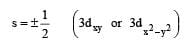which is possible only for one e.

QUESTION: 2

### Which of the following is isoelectronic? 

Solution:

Both CN and CO have 14 electrons

QUESTION: 3

### In hydrogen atom, energy of first excited state is –3.4 eV. Find out KE of the same orbit of Hydrogen atom 

Solution:

Suppose the nucleus of hydrogen atom have charge of one proton i.e. The electron revolves in a radius of r around it. Therefore the centripital force is supplied by electrostatic force of attraction i.e.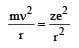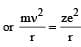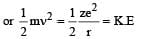now total energy (En) = K.E + P.E in first excited state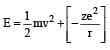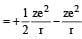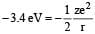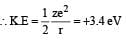QUESTION: 4

The value of Planck's constant is 6.63 × 10–34 Js.The velocity of light is 3.0 × 108 m s–1. Which value is closest to the wavelength in nanometers of a quantum of light with frequency of 8 × 1015 s–1 ? 

Solution: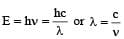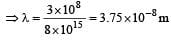In nanometer λ = 3.75× 10 which is closest to 4 × 101

QUESTION: 5

The ions O2–, F, Na+, Mg2+ and Al3+ are isoelectronic. Their ionic radii show 

Solution:

Amongst isoelectronic species, ionic radii of anion is more than that of cations. Further size of anion increase with increase in-ve change and size of cation decrease with increase in + ve charge. Hence ionic radii decreases from O to Al+++.

QUESTION: 6

The frequency of radiation emitted when the electron falls from n = 4 to n = 1 in a hydrogen atom will be (Given ionization energy of H=2.18 ×10–18J atom–1and h = 6.625 × 10–34 J s ) 

Solution: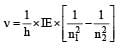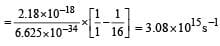QUESTION: 7

The energy of the second Bohr orbit of the hydrogen atom is -328 kJ mol-1; hence the energy of the fourth Bohr orbit would be: 

Solution:

We know that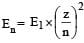Given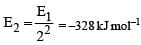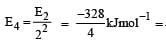–82 kJmol–1

QUESTION: 8

Given : The mass of electron is 9.11 × 10–31 kg Plank constant is 6.626 × 10–34 Js, the uncertainty involved in the measurement of velocity within a distance of 0.1 Å is 

Solution:

We know that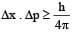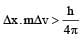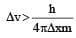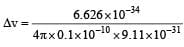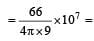5.79 x106 m/sec

QUESTION: 9

The orientation of an atomic orbital is governed by 

Solution:

Magnetic quantum no. represents the orientation of atomic orbitals in an atom. For example px, py & pz have orientation along X-axis, Y-axis & Z-axis

QUESTION: 10

Consider the following sets of quantum numbers: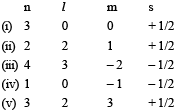Which of the following sets of quantum number is not possible? 

Solution:

(ii) is not possible for any value of n because l varies from 0 to (n – 1) thus for n = 2, l can be only 0, 1, 2. (iv) is not possible because for l = 0, m = 0. (v) is not possible because for l = 2, m varies from –2 to +2.

QUESTION: 11

If uncertainty in position and momentum are equal, then uncertainty in velocity is : 

Solution:

We know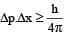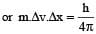[∵ Δp= mΔv]

since Δp = Δx (given)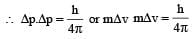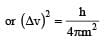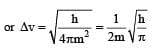Thus option (a) is the correct option.

QUESTION: 12

The measurement of the electron position if associated with an uncertainty in momentum, which is equal to 1 × 10–18 g cm s– 1 . The uncertainty in electron velocity is? (mass of an electron is 9 × 10– 28 g)


Solution:

Δp = mΔv Substituting the given values of Δx and m, we get 1×10–18 gcms–1 = 9×10–28g × Δv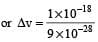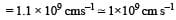i.e. option (a) is correct.

QUESTION: 13

The energy absorbed by each molecule (A2) of a substance is 4.4 × 10–19 J and bond energy per molecule is 4.0 × 10–19 J. The kinetic energy of the molecule per atom will be: 

Solution:

K.E per atom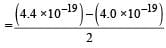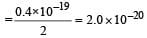QUESTION: 14

Maximum number of electrons in a subshell of an atom is determined by the following: 

Solution:

The number of sub shell is (2 l + 1). The maximum number of electrons in the sub shell is 2 (2 l + 1) = (4 l + 2)

QUESTION: 15

Wh ich of the followin g is not per missible arrangement of electrons in an atom? 

Solution:

m = 2 l +1, thus for l  = 2, m = 5, hence values of m will be – 2, –1, 0, + 1, + 2.
Therefore for l = 2, m cannot have the value– 3.

QUESTION: 16

The electronic configuration for oxygen is written as 1s22s22p4. Which rule will this configuration be violating?

Solution:
QUESTION: 17

Which one of the following species does not exist under normal conditions? 

Solution:

Bond order of Be2 = 0, hence Be2 cannot exist.

QUESTION: 18

A 0.66 kg ball is moving with a speed of 100 m/s.The associated wavelength will bexh (6.6x10-34 Js) : 

Solution: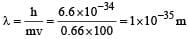QUESTION: 19

The total number of atomic orbitals in fourth energy level of an atom is : 

Solution:

Total no. of atomic orbital in a shell = n2.
Given n = 4; Hence number of atomic orbitals in 4th shell will be 16.

QUESTION: 20

Which of the following has the minimum bond length ? 

Solution: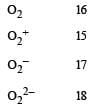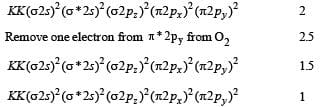Since, the bond length decreases as the bond order increases, hence, O2+ have least bond length.

QUESTION: 21

The energies E1 and E2 of two radiations are 25 eV and 50 eV, respectively. The relation between their wavelengths i.e., λ1 and λ2 will be :

Solution:

Given E1 = 25eV E2 = 50 eV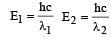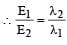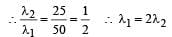QUESTION: 22

If n = 6, the correct sequence for filling of electrons will be : 

Solution:

ns → (n - 2)f → (n - 1)d → np [n = 6]

QUESTION: 23

According to the Bohr Theory, which of the following transitions in the hydrogen atom will give rise to the least energetic photon ? [2011 M]

Solution:

Energy of photon obtained from the transition n = 6 to n = 5 will have least energy.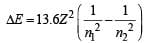QUESTION: 24

The pairs of species of oxygen and their magnetic behaviours are noted below. Which of the following presents the correct description? [2011 M]

Solution:

MOT configurations of O2 and O2+ :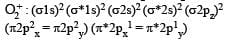Number of unpaired electrons = 2, so paramagnetic.

O2 : (σ1s)2 (σ*1s)2 (σ2s)2 (σ*2s)2 (σ2pz)2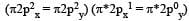Number of unpaired electrons = 1, so paramagnetic.

QUESTION: 25

Maximum number of electrons in a subshell with : l = 3 and n = 4 is : 

Solution:

(n = 4, l = 3) ⇒  4f subshell Since, maximum no. of electrons in a subshell = 2(2l + 1)
So, total no. of electron in 4f subshell = 2 (2 × 3 + 1) = 14 electrons.

QUESTION: 26

The correct set of four quantum numbers for the valence electron of rubidium atom (Z = 37) is 

Solution:

Electronic configuration of  Rb = [Kr] 5s–1 Set of quantum numbers,  n = 5
ℓ = 0, ∴ s orbital  m  =  0, s = + 1/2

QUESTION: 27

The orbital angular momentum of a p-electron is given as : [2012 M]

Solution:

Orbital angular momentum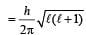For p orbital ℓ = 1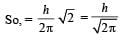QUESTION: 28

The value of Planck’s constant is 6.63 × 10–34 Js.The speed of light is 3 × 1017 nm s–1.. Which value is closest to the wavelength in nanometer of a quantum of light with frequency of 6 × 1015 s–1? [NEET 2013]

Solution:

C = νλ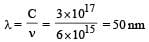QUESTION: 29

What is the maximum numbers of electrons that can be associated with the following set of quantum numbers? n = 3, l = 1 and m = –1 [NEET 2013]

Solution:

n = 3 → 3rd shell
l = 1 → p sub shell.
m = – 1 is possible for two electrons present in an orbital.

QUESTION: 30

Based on equation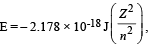certain conclusions are written. Which of them is not correct ? [NEET 2013]

Solution:

Energy of an electron at infinite distance from the nucleus is zero. As an electron approaches the nucleus, the electron attraction increases and hence the energy of electron decreases and thus becomes negative. Thus as the value of n decreases, i.e. lower the orbit is, more negative is the energy of the electron in it.

QUESTION: 31

According to law of photochemical equivalence the energy absorbed (in ergs/mole) is given as (h = 6.62 × 10–27 ergs,  c = 3 × 1010 cm s–1, NA = 6.02 × 1023 mol–1) [NEET Kar. 2013]

Solution:

(N)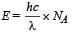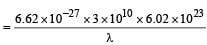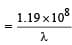Hence none of the given option is correct.Use Code STAYHOME200 and get INR 200 additional OFF Use Coupon Code

### How to Prepare for NEET

Read our guide to prepare for NEET which is created by Toppers & the best Teachers